# Electronics and Communication Engineering - Networks Analysis and Synthesis

1.

If P = 250 W and p.f. = 0.707 lagging, Q =

 A. 250 vars B. - 250 vars C. 176.7 vars D. - 176.75 vars

Explanation:

Apparent power =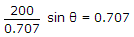, Q = 250 vars and lagging vars are taken as positive.

2.

Figure shows an R-L circuit. The current is i = 1 - e-t. The rate of change of current at t = 1 second is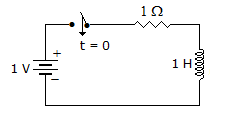A. 1 A/s B. 0.63 A/s C. 0.37 A/s D. 0

Explanation: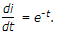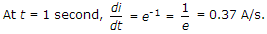3.

Assertion (A): Energy stored in inductance is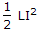and that in capacitance is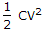Reason (R): Inductance and capacitance are dual of each other.

 A. Both A and R are true and R is correct explanation of A B. Both A and R are true and R is not the correct explanation of A C. A is true but R is false D. A is false but R is true

Explanation:

In principle of duality L is dual of C and I is dual of V.

4.

The time constant of the circuit after the switch shown in the figure is opened is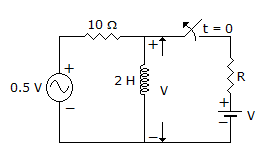A. 0.2 s B. 5 s C. 0.1 s D. dependent on R and hence cannot be determined unless R is known

Explanation: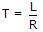.

5.

A series RLC circuit is at resonance at 200 Hz. If capacitance is increased to four times, the circuit will be in resonance at

 A. 200 Hz B. 400 Hz C. 100 Hz D. 800 Hz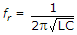. If C becomes four times, f is reduced to half.